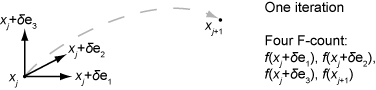## Iterations and Function Counts

In general, Optimization Toolbox™ solvers iterate to find an optimum. A solver begins at an initial value x0, performs some intermediate calculations that eventually lead to a new point x1, and then repeats the process to find successive approximations x2, x3, ... of the local minimum. Processing stops after some number of iterations k.

You can limit the number of iterations or function counts by setting the `MaxIterations` or `MaxFunctionEvaluations` options for a solver using `optimoptions`. Or, if you want a solver to continue after reaching one of these limits, raise the values of these options. See Set and Change Options.

At any step, intermediate calculations can involve evaluating the objective function and any constraints at points near the current iterate xi. For example, the solver might estimate a gradient by finite differences. At each nearby point, the function count (`F-count`) increases by one. The figure Typical Iteration in 3-D Space shows that, in 3-D space with forward finite differences of size delta, one iteration typically corresponds to an increase in function count of four. In the figure, ei represents the unit vector in the ith coordinate direction.

Typical Iteration in 3-D Space• If the problem has no constraints, the `F-count` reports the total number of objective function evaluations.

• If the problem has constraints, the `F-count` reports only the number of points where function evaluations took place, not the total number of evaluations of constraint functions. So, if the problem has many constraints, the `F-count` can be significantly less than the total number of function evaluations.

Sometimes a solver attempts a step and rejects the attempt. The `trust-region`, `trust-region-reflective`, and `trust-region-dogleg` algorithms count these failed attempts as iterations, and report the (unchanged) result in the iterative display. The `interior-point`, `active-set`, and `levenberg-marquardt` algorithms do not count failed attempts as iterations, and do not report the attempts in the iterative display. All attempted steps increase the `F-count`, regardless of the algorithm.

`F-count` is a header in the iterative display for many solvers. For an example, see Interpret Result.

The `F-count` appears in the output structure as `output.funcCount`, enabling you to access the evaluation count programmatically. For more information, see Output Structures.